# McGraw Hill Math Grade 3 Chapter 5 Lesson 2 Answer Key Dividing by 3

## McGraw-Hill Math Grade 3 Answer Key Chapter 5 Lesson 2 Dividing by 3

Solve.

Divide. Use repeated subtraction or objects to help.

Question 1.
21 ÷ 3 = ___
21 ÷ 3 = 7

Explanation:
Use Repeated subtraction:
21 – 3 = 18, 18 – 3 = 15, 15 – 3 = 12, 12 – 3 = 9, 9 – 3 = 6, 6 – 3 = 3 and 3 – 3 = 0
I subtracted 21 with 3 seven times
So, 21 ÷ 3 = 7.

Question 2.
9 ÷ 3 = ___
9 ÷ 3 = 3

Explanation:
Use Repeated subtraction:
9 – 3 = 6, 6 – 3 = 3 and 3 – 3 = 0
I subtracted 9 with 3 three times
So, 9 ÷ 3 = 3.

Question 3.
15 ÷ 3 = ___
15 ÷ 3 = 5

Explanation:
Use Repeated subtraction:
15 – 3 = 12, 12 – 3 = 9, 9 – 3 = 6, 6 – 3 = 3 and 3 – 3 = 0
I subtracted 15 with 3 five times
So, 15 ÷ 3 = 5.

Question 4.
0 ÷ 3 = ___
0 ÷ 3 = 0

Explanation:
Use Repeated subtraction:
Zero divided by any number is 0
So, 0 ÷ 3 = 0.

Question 5.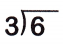Explanation:
6 ÷ 3 = 2
Six divided by three is two.

Question 6.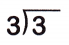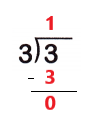Explanation:
3 ÷ 3 = 1
Three divided by three is one.

Question 7.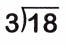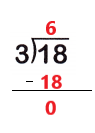Explanation:
18 ÷ 3 = 6
Eighteen divided by three is six.

Question 8.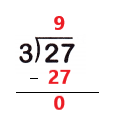Explanation:
27 ÷ 3 = 9
Twenty seven divided by three is nine.

Question 9.
Ava is putting 24 baseball cards in an album. Each page can hold 3 baseball cards. How many pages can Ava fill?
____ ÷ ____ = ___ pages